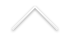Título: Cutoff for the Glauber dynamics of the discrete Gaussian free field

Palestrante: Reza Gheissari, UC Berkeley
Data: 22/09/2021
Horário: 13:00h
Local: Transmissão online

Confira AQUI o link para a transmissão.

Resumo: The Gaussian free field (GFF) is a canonical model of random surfaces in probability theory, generalizing the Brownian bridge to higher dimensions. It arises naturally as the stationary solution to the stochastic heat equation with additive noise (SHE), and together the SHE and GFF are expected to be the universal scaling limit of the dynamics and equilibrium of many random surface models arising in lattice statistical physics. We study the mixing time (time to converge to stationarity, when started out of equilibrium) for the central pre-limiting object, the discrete Gaussian free field (DGFF) evolving under the Glauber dynamics. In joint work with S. Ganguly, we establish that for every dimension d larger than one , on a box of side-length n in Zd, the Glauber dynamics for the DGFF exhibits cutoff at time (d/\pi^2) n^2 \log n with an O(n^2) window. Our proof relies on an "exact" representation of the DGFF dynamics in terms of random walk trajectories with space-dependent jump times, which we expect to be of independent interest.

ID da reunião: 958 0581 3232